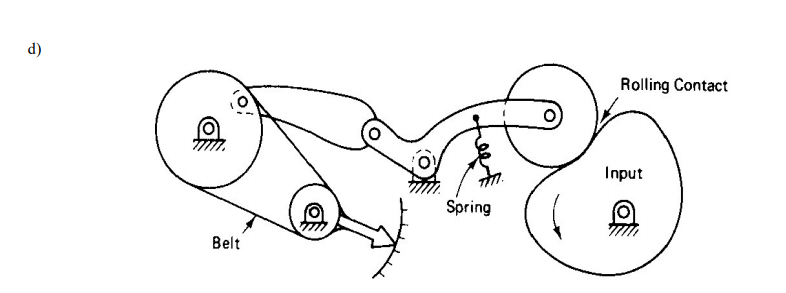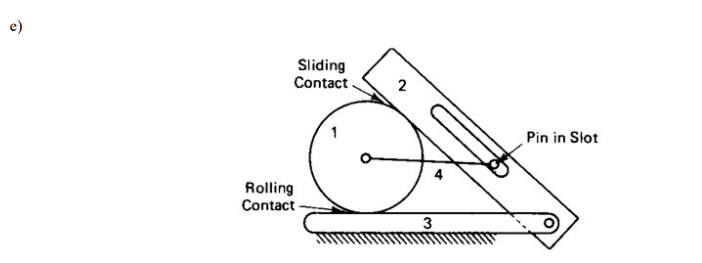# Draw the kinematic diagram of linkage and determine the degrees of freedom for each mechanism, use the equation and indicate the number of members and joints clearly

Question-AnswerCategory: Kinematics of MachineDraw the kinematic diagram of linkage and determine the degrees of freedom for each mechanism, use the equation and indicate the number of members and joints clearlyDraw the kinematic diagram of linkage and determine the degrees of freedom for each mechanism, use the equation and indicate the number of members and joints clearly